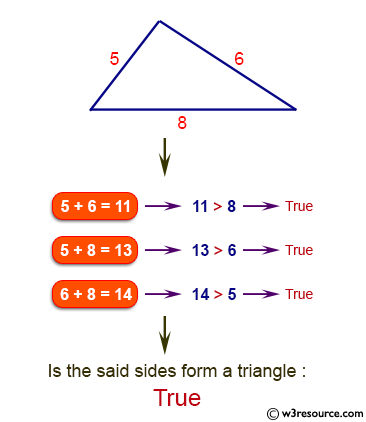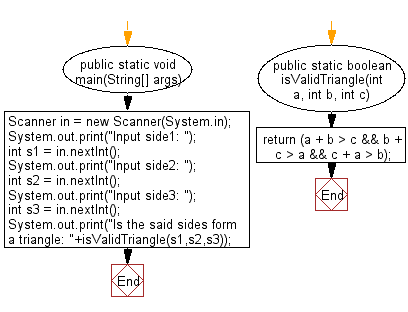# Java Exercises: Check if three given side lengths can make a triangle or not

## Java Basic: Exercise-195 with Solution

Write a Java program to check if three given side lengths (integers) can make a triangle or not.

Pictorial Presentation:Sample Solution:

Java Code:

``````import java.util.*;
public class Solution {
public static void main(String[] args) {
Scanner in = new Scanner(System.in);
System.out.print("Input side1: ");
int s1 = in .nextInt();
System.out.print("Input side2: ");
int s2 = in .nextInt();
System.out.print("Input side3: ");
int s3 = in .nextInt();

System.out.print("Is the said sides form a triangle: " + isValidTriangle(s1, s2, s3));
}
public static boolean isValidTriangle(int a, int b, int c) {
return (a + b > c && b + c > a && c + a > b);
}
}
```
```

Sample Output:

```Input side1:  5
Input side2:  6
Input side3:  8
Is the said sides form a triangle: true
```

Flowchart:Java Code Editor:

Company:

What is the difficulty level of this exercise?

﻿

## Java: Tips of the Day

Parsing dates:

```import java.io.*;
import java.util.*;
import java.text.*;

String s = "2001/09/23 14:39";

SimpleDateFormat formatter = new SimpleDateFormat ("yyyy/MM/dd H:mm");
Date d = formatter.parse(s, new ParsePosition(0));
```